# Excel CEILING Function

This post will guide you how to use Excel CEILING function with syntax and examples in Microsoft excel.

### Description

The Excel CEILING function returns a given number rounded up to the nearest multiple of a given number of significance. So you can use the CEILING function to round up a number to the nearest multiple of a given number.

The CEILING function is a build-in function in Microsoft Excel and it is categorized as a Math and Trigonometry Function.

The CEILING function is available in Excel 2016, Excel 2013, Excel 2010, Excel 2007, Excel 2003, Excel XP, Excel 2000, Excel 2011 for Mac.

### Syntax

The syntax of the CEILING function is as below:

`= CEILING (number, significance)`

Where the CEILING function arguments are:

• number – This is a required argument. The number that you want to round up.
• significance – This is a required argument. The multiple of significance to which you want to round a number to.

Note:

• If either number and significance arguments are non-numeric, the CEILING function will return the #VALUE! Error.
• If the number argument is negative, and the significance argument is also negative, the number is rounded down, and it will be away from 0.
• If the number argument is negative and the significance is positive, the number is rounded up towards zero.

### Excel CEILING Function Examples

The below examples will show you how to use Excel CEILING Function to round a number up to nearest multiple.

1# to round 4.6 up to nearest multiple of 3, enter the following formula in Cell B1.

`=CEILING(4.6,3)`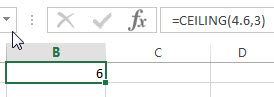2# to round -4.6 up to nearest multiple of -3, enter the following formula in Cell B2.

`=CEILING(-4.6,-3)`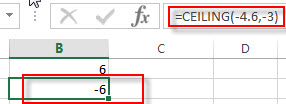3# to round -4.6 up to nearest multiple of 2, enter the following formula in Cell B3.

`=CEILING(-4.6,2)`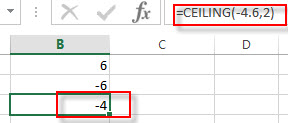4# to round 2.6 up to nearest multiple of 0.2, enter the following formula in Cell B4.

`=CEILING(2.6,0.2)`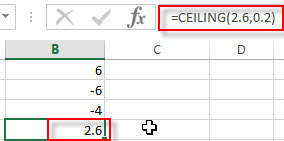5# to round 0.26 up to nearest multiple of 0.02, enter the following formula in Cell B5.

`=CEILING(0.26,0.02)`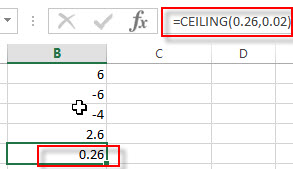### Related Functions

• Excel ROUNDDOWN function
The Excel ROUNDDOWN function round the number down to the specified number of digits. The syntax of the ROUNDDOWN function is as below:=ROUNDDOWN (number, num_digits)…
• Excel ROUND function
The Excel ROUND function rounds a number to a specified number of digits. You can use the ROUND function to round to the left or right of the decimal point in Excel.The syntax of the ROUND function is as below:=ROUND (number, num_digits)…
• Excel ROUNDUP function
The Excel ROUNDUP function rounds the number up to a specified number of decimal places. It will round away from 0.The syntax of the ROUNDUP function is as below:=ROUNDUP (number, num_digits)…